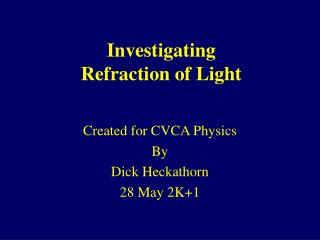DownloadDownload PresentationInvestigating Refraction of Light

# Investigating Refraction of Light

Download Presentation## Investigating Refraction of Light

- - - - - - - - - - - - - - - - - - - - - - - - - - - E N D - - - - - - - - - - - - - - - - - - - - - - - - - - -
##### Presentation Transcript

1. InvestigatingRefraction of Light Created for CVCA Physics By Dick Heckathorn 28 May 2K+1

2. Table of Contents 2 Light from Air into Plastic 8 Light from Plastic into Air 15 Determine Critical Angle 18 Light through a Prism

3. Refraction Light traveling from air into plastic.

4. Refraction N Air 1.00 Plastic 1.51

5. Refraction Air 1.00 Plastic 1.51 Calculate angle r

6. Refraction Air 1.00 Plastic 1.51 Calculate angle r

7. Refraction Air 1.00 Plastic 1.51 Calculate angle r

8. Refraction Air 1.00 Plastic 1.51 Calculate angle r

9. Refraction Light traveling from plastic into air.

10. Refraction Air 1.00 Plastic 1.51 Calculate angle r

11. Refraction Air 1.00 Plastic 1.51 Calculate angle r

12. Refraction Air 1.00 Plastic 1.51 Calculate angle r

13. Refraction Air 1.00 Plastic 1.51 Calculate angle r

14. Refraction Air 1.00 Calculate angle r Calculator says ? All is reflected No refraction Plastic 1.51

15. Refraction Air 1.00 Calculate angle r Calculator says? Plastic 1.51

16. Finding the Critical Angle What will be the angle in plastic when the angle in air is 900

17. Finding the Critical Angle N Air 1.00 Plastic 1.51

18. Finding the Critical Angle

19. Measure angle i Draw ray i Air 1.00 Draw ray r Calculate angle r 60o Draw ray r Find new angle i Calculate angle r Plastic 1.51 60o 60o

20. Refraction Air 1.00 Plastic 1.51

21. Refraction Air 1.00 Plastic 1.51

22. Refraction Air 1.00 Plastic 1.51

23. Refraction Air 1.00 Plastic 1.51

24. Refraction Air 1.00 Plastic 1.51

25. Refraction Air 1.00 Plastic 1.51

26. Refraction Air 1.00 Plastic 1.51

27. Refraction Air 1.00 Plastic 1.51

28. Refraction Air 1.00 Plastic 1.51

29. Refraction Air 1.00 Plastic 1.51